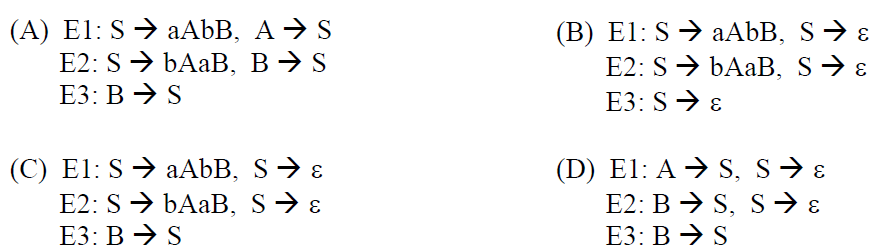Related Articles
GATE | GATE CS 2012 | Question 65
• Last Updated : 30 Nov, 2013

Consider the date same as above question. The appropriate entries for E1, E2, and E3 are(A) A
(B) B
(C) C
(D) D

Explanation:

As we need to find entries E1, E2 and E3 which are against Non terminals S and B, so we will deal with only those productions which have S and B on LHS.

Here representing the parsing table as M[ X , Y ], where X represents rows( Non terminals) and Y represents columns(terminals).

```
Rule 1: if P --> Q is a production, for each terminal
't' in FIRST(Q) add P-->Q to M [ P , t ]

Rule 2 : if epsilon is in FIRST(Q), add P --> Q to
M [ P , f ] for each f in FOLLOW(P). ```

For the production rule S–> aAbB, it will be added to parsing table at the position M[ S , FIRST( aAbB ) ], Now FIRST(aAbB) = {a}, hence add S–> aAbB to M[ S , a ] which is E1.

For the production rule S–> bAaB, it will be added to parsing table at the position M[ S , FIRST( bAaB ) ], Now FIRST(bAaB) = {b}, hence add S–> bAaB to M[ S , b ] which is E2

For the production rule S–> epsilon , it will be added to parsing table at the position M[ S , FOLLOW(S) ], Now FOLLOW(S) = { a , b , \$ }, hence add S –> epsilon to M[ S , a ] and M[ S , b ] which are again E1 and E2 respectively.

For the production rule B –> S, it will be added to parsing table at the position M[ B , FIRST( S ) ], Now FIRST(S) also contains epsilon, hence add B –> S to M[ S , FOLLOW(B) ], and FOLLOW(B) contains {\$}, i.e. M[ S , \$ ] which is E3.

epsilon corresponds to empty string.

Quiz of this Question

My Personal Notes arrow_drop_up